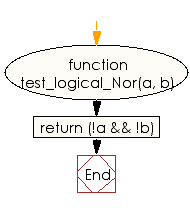# JavaScript: Create the value of NOR of two given booleans

## JavaScript Basic: Exercise-124 with Solution

Write a JavaScript program to create the value of NOR of two given booleans.

Note: In boolean logic, logical nor or joint denial is a truth-functional operator which produces a result that is the negation of logical or. That is, a sentence of the form (p NOR q) is true precisely when neither p nor q is true - i.e. when both of p and q are false

Sample Example:

For x = true and y = false, the output should be logical_Nor(x, y) = false; For x = false and y = false, the output should be logical_Nor(x, y) = true.

Sample Solution:

HTML Code:

``````<!DOCTYPE html>
<html>
<meta charset="utf-8">
<meta name="viewport" content="width=device-width">
<title> Create the value of NOR of two given booleans</title>
<body>

</body>
</html>
```
```

JavaScript Code:

``````function test_logical_Nor(a, b) {
return (!a && !b)
}
console.log(test_logical_Nor(true, false));
console.log(test_logical_Nor(false, false));
console.log(test_logical_Nor(true, true));
``````

Sample Output:

```false
true
false
```

Flowchart:ES6 Version:

``````function test_logical_Nor(a, b) {
return (!a && !b)
}
console.log(test_logical_Nor(true, false));
console.log(test_logical_Nor(false, false));
console.log(test_logical_Nor(true, true));
``````

Live Demo:

See the Pen javascript-basic-exercise-124 by w3resource (@w3resource) on CodePen.

Improve this sample solution and post your code through Disqus

What is the difficulty level of this exercise?

Test your Programming skills with w3resource's quiz.

﻿

## JavaScript: Tips of the Day

Returns the sum of the powers of all the numbers from start to end (both inclusive)

Example:

```const sumPower = (end, power = 2, start = 1) =>
Array(end + 1 - start)
.fill(0)
.map((x, i) => (i + start) ** power)
.reduce((a, b) => a + b, 0);
console.log(sumPower(10)); // 385
console.log(sumPower(10, 3)); // 3025
console.log(sumPower(10, 3, 5)); // 2925
```

Output:

```385
3025
2925
```